## Tamilnadu Samacheer Kalvi 12th Maths Solutions Chapter 6 Applications of Vector Algebra Ex 6.6

Question 1.
Find a parametric form of vector equation of a plane which is at a distance of 7 units from the origin having 3, -4, 5 as direction ratios of a normal to it.
Solution:
Given p = 7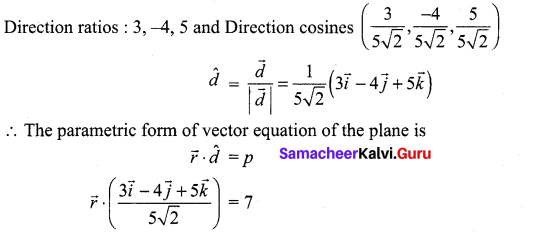Question 2.
Find the direction cosines of the normal to the plane 12x + 3y – 4z = 65. Also, find the non-parametric form of vector equation of a plane and the length of the perpendicular to the plane from the origin.
Solution:
12x + 3y – 4z = 65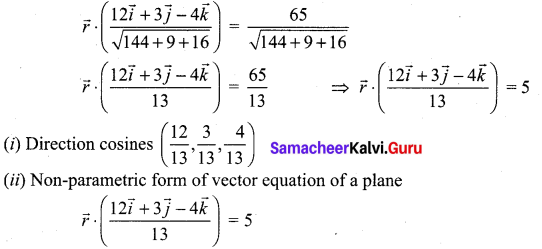(iii) Length of the perpendicular to the plane from the origin is 5 units.Question 3.
Find the vector and Cartesian equation of the plane passing through the point with position vector $$2 \hat{i}+6 \hat{j}+3 \hat{k}$$ and normal to the vector $$\hat{i}+3 \hat{j}+5 \hat{k}$$
Solution: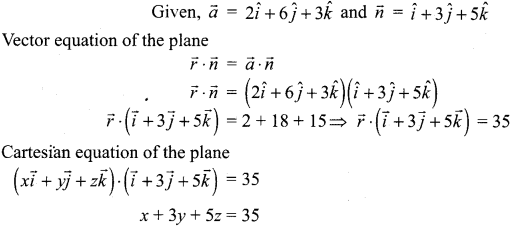Question 4.
A plane passes through the point (-1, 1, 2) and the normal to the plane of magnitude $$3 \sqrt{3}$$ makes equal acute angles with the coordinate axes. Find the equation of the plane.
Solution:
Given magnitude = $$3 \sqrt{3}$$ and $$\vec{a}=-\vec{i}+\vec{j}+2 \vec{k}$$
Then, the normal vector makes equal acute angle with the coordinate axes.
We know that cos2 α + cos2 β + cos2 γ = 1 (But α = β = γ)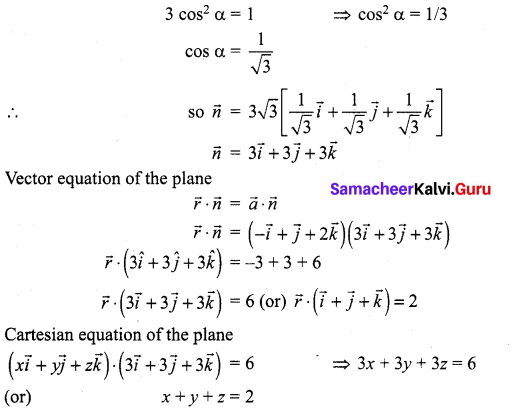Question 5.
Find the intercepts cut off by the plane $$\vec{r} \cdot(6 \hat{i}+4 \hat{j}-3 \hat{k})$$ = 12 on the coordinate axes.
Solution: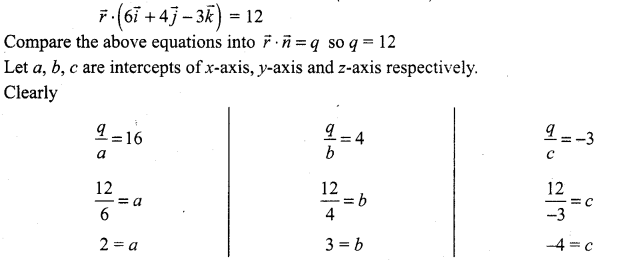x-intercept = 2; y-intercept = 3; z-intercept = -4

Question 6.
If a plane meets the coordinate axes at A, B, C such that the centroid of the triangle ABC is the point (u, v, w), find the equation of the plane.
Solution:
Let A (a, 0, 0), B(0, b, 0), C(0, 0, c)
centroid of ∆ABC = $$\left(\frac{a}{3}, \frac{b}{3}, \frac{c}{3}\right)$$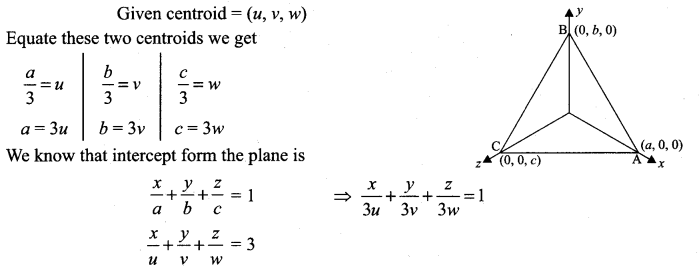### Samacheer Kalvi 12th Maths Solutions Chapter 6 Applications of Vector Algebra Ex 6.6 Additional Problems

Question 1.
Find the vector and cartesian equations of a plane which is at a distance of 18 units from the origin and which is normal to the vector $$2 \vec{i}+7 \vec{j}+8 \vec{k}$$
Solution:Question 2.
Find the unit vector to the plane 2x – y + 2z = 5.
Solution:
Writing the plane in normal form we get,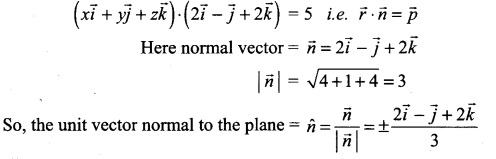Question 3.
Find the length of the perpendicular from the origin to the plane $$\vec{r} \cdot(3 \vec{i}+4 \vec{j}+12 \vec{k})$$ = 26.
Solution:
Taking the equation of the plane in cartesian form we get,
$$(x \vec{i}+y \vec{j}+z \vec{k}) \cdot(3 \vec{i}+4 \vec{j}+12 \vec{k})$$ = 26
i.e., 3x + 4y+ 12z – 26 = 0
The length of the perpendicular from (0, 0, 0) to the above plane is
$$\pm \frac{-26}{\sqrt{9+16+144}}=\frac{+26}{13}$$ = 2 units

Question 4.
The foot of the perpendicular drawn from the origin to a plane is (8, -4, 3). Find the equation of the plane.
Solution:
The required plane passes through the point A(8, -4, 3) and is perpendicular to $$\overrightarrow{\mathrm{OA}}$$ .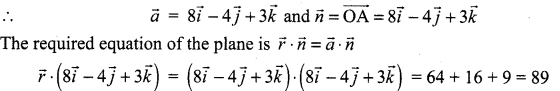The cartesian equation is 8x – 4y + 3z = 89.

Question 5.
Find the equation of the plane through the point whose position vector is $$2 \vec{i}-\vec{j}+\vec{k}$$ and perpendicular to the vector $$4 \vec{i}+2 \vec{j}-3 \vec{k}$$.
Solution:
The required plane is perpendicular to $$4 \vec{i}+2 \vec{j}-3 \vec{k}$$
So, it is parallel to the plane 4x + 2y – 3z = k
∴ the equation of the plane is 4x + 2y – 3z = k
The plane passes through the point (2, -1, 1)
⇒ (4)(2) + 2(-1) – 3(1) = λ i.e. λ = 8 – 2 – 3 = 3
So, the equation of the plane is 4x + 2y – 3z = 3.Question 6.
Find the vector and cartesian equations of the plane passing through the point (2, -1, 4) and parallel to the plane $$\vec{r} \cdot(4 \vec{i}-12 \vec{j}-3 \vec{k})$$ = 7.
Solution:
The given plane is $$\vec{r} \cdot(4 \vec{i}-12 \vec{j}-3 \vec{k})$$ = 7
i e. $$(x \vec{i}+y \vec{j}+z \vec{k}) \cdot(4 \vec{i}-12 \vec{j}-3 \vec{k})$$ = 7
i.e. 4x – 12y – 3z = 1
The required plane is parallel to the above plane. So, the equation of the required plane is 4x – 12y – 3z – k. The plane passes through (2, -1, 4).
⇒ 4(2) – 12(-1) – 3(4) = k i.e. k = 8 + 12 – 12 = 8
So, the equation of the plane is 4x – 12y – 3z = 8.